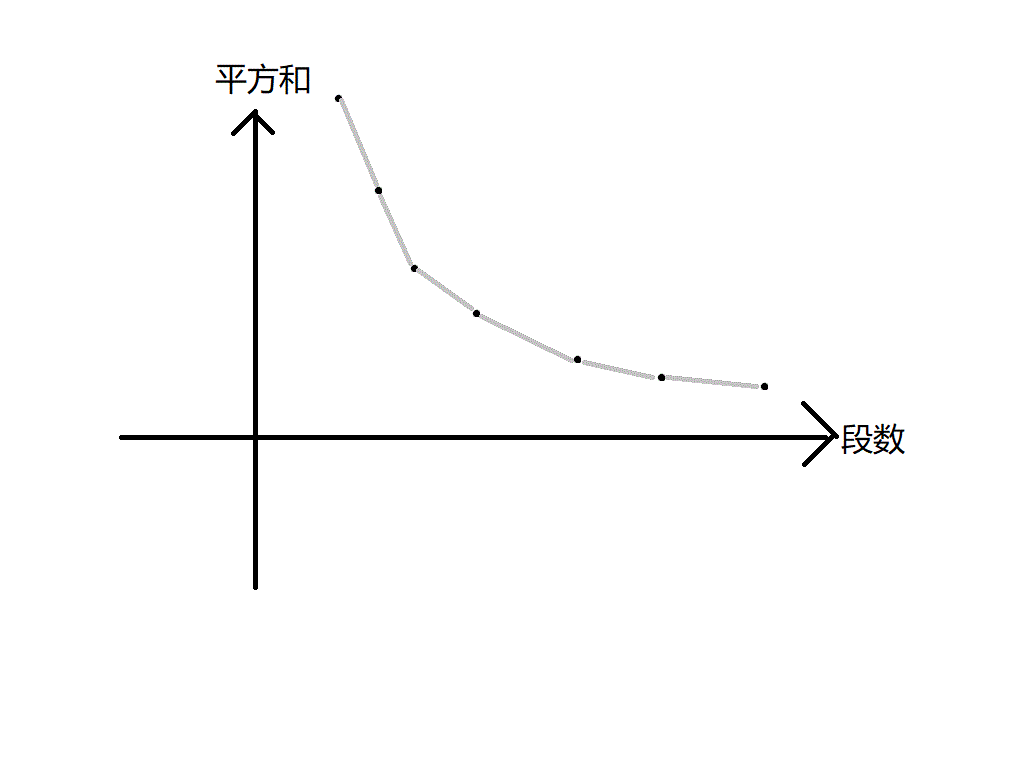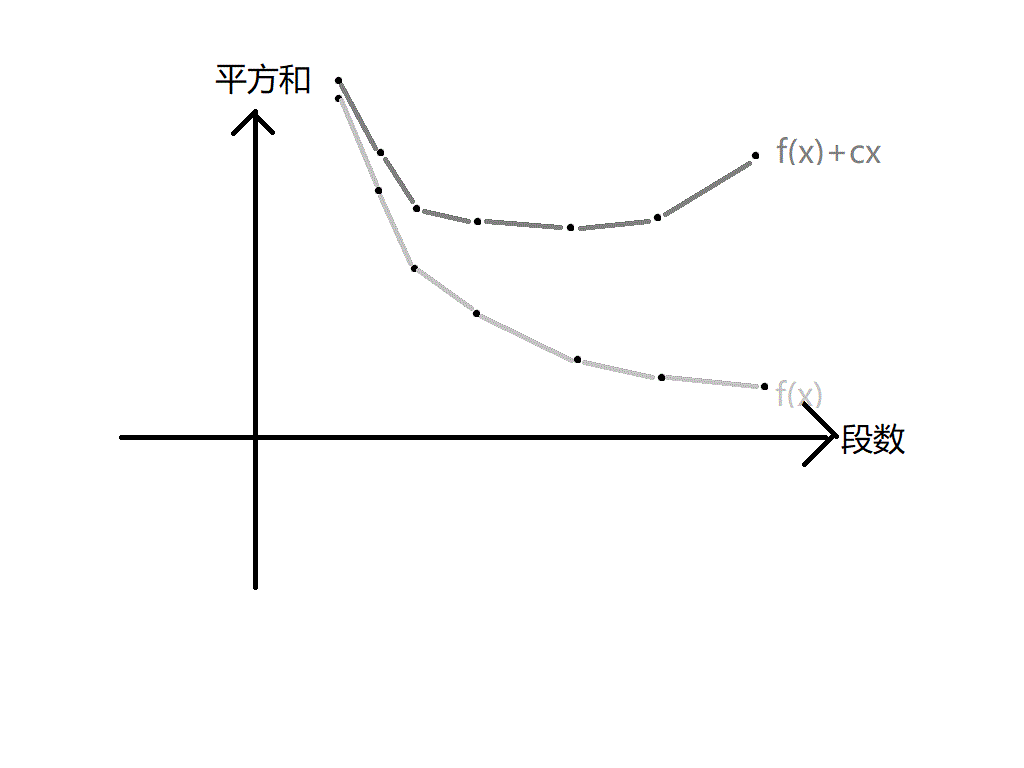# 例题

### 朴素做法

$$f[i][j]=min(f[u][j-1]+(S[i]-S[u])^2)$$

# 理性证明

• 我们有可能没法通过改变附加权值使得分的段数等于 k，因为有可能段数关于附加权值的函数不连续。
• 我们还没有证明通过改变附加权值求得的解一定是最优解。

### 将问题函数化### 总结一下

• 1: $x=x_0$，那么答案就是：$y-x_0\cdot c$
• 2: $x<x_0$，那么答案也是：$y-x_0\cdot c$

# 一些疑问

Update:

### 4 条评论